# 4. (15 pts Consider the following direction fields IV VI (5 pts)Which of the direction fields corresponds to the system x -Ax, where A is a 2x2 matrix with eigenvalues λ,--1 and λ2-2 and correspondin...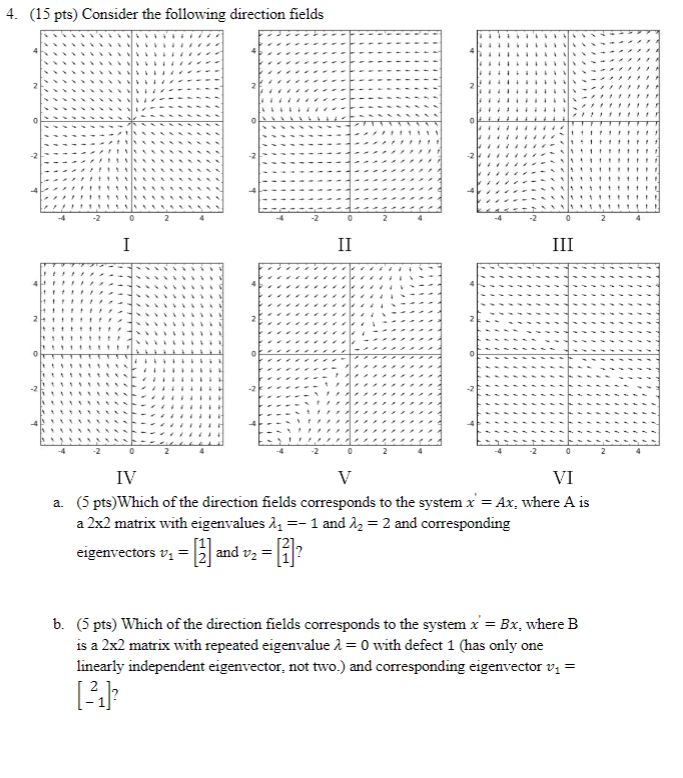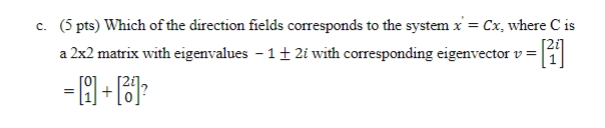4. (15 pts Consider the following direction fields IV VI (5 pts)Which of the direction fields corresponds to the system x -Ax, where A is a 2x2 matrix with eigenvalues λ,--1 and λ2-2 and corresponding eigenvectors vand v- 1? a. is a 2x2 matrix with repeated eigenvalue λ = 0 with defect 1 (has only one linearly independent eigenvector, not two.) and corresponding eigenvector vi- 13
(5 pts) Which of the direction fields corresponds to the system x -Cx, where C is a 2x2 matrix with eigenvalues-l t 2i with corresponding eigenvector v c. 01 [2i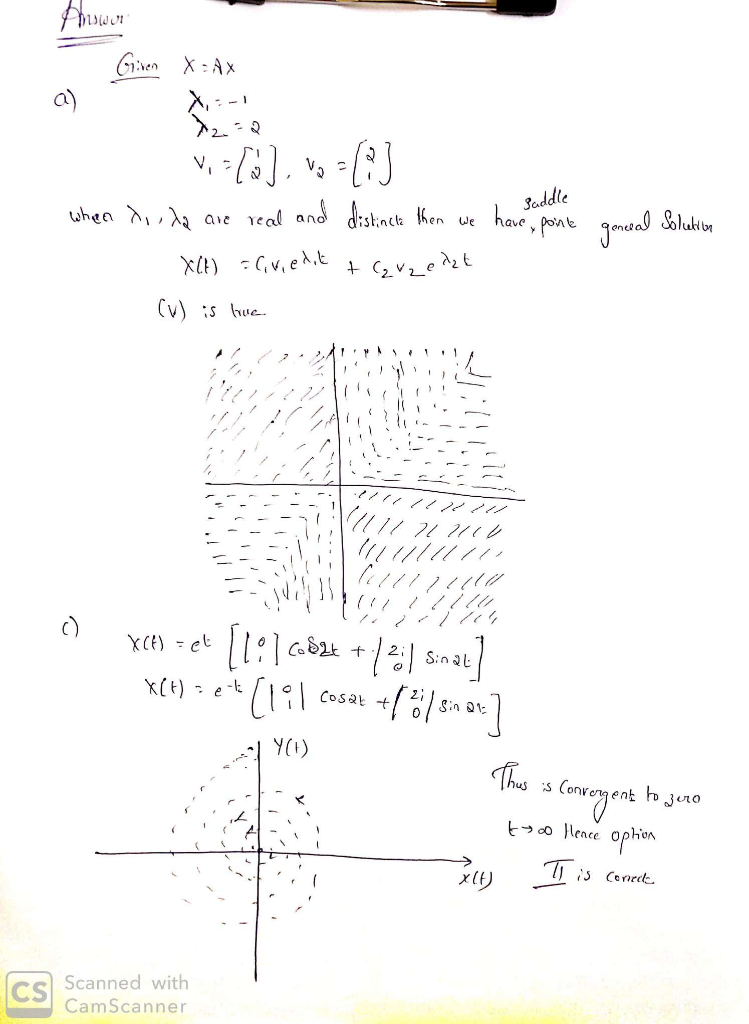##### Add Answer of: 4. (15 pts Consider the following direction fields IV VI (5 pts)Which of the direction fields corresponds to the system x -Ax, where A is a 2x2 matrix with eigenvalues λ,--1 and λ2-2 and correspondin...
Similar Homework Help Questions
• ### 5. The following matrix B has known eigenvalues λ1-1 and λ2-6. 10a-1 B-0b-23 c30 0 Where...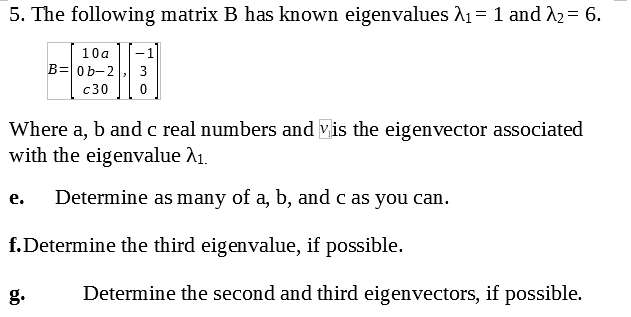5. The following matrix B has known eigenvalues λ1-1 and λ2-6. 10a-1 B-0b-23 c30 0 Where a, b and c real numbers and vis the eigenvector associated with the eigenvalue A1. e. Determine as many of a, b, and c as you can. f.Determine the third eigenvalue, if possible. g.Determine the second and third eigenvectors, if possible.

• ### Consider the 2×22×2 matrix AA given by A=1−2[−5−1−1−5].A=1−2[−5−1−1−5].. Find the eigenvalues λ+λ+ and λ−λ−, larger and...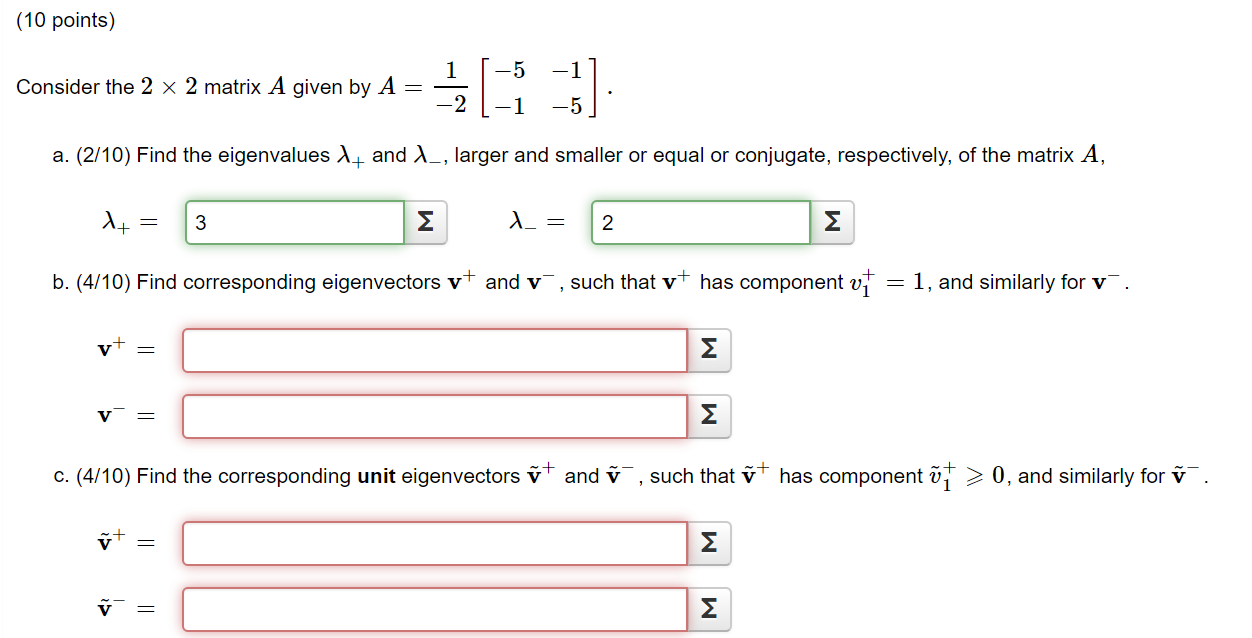Consider the 2×22×2 matrix AA given by A=1−2[−5−1−1−5].A=1−2[−5−1−1−5].. Find the eigenvalues λ+λ+ and λ−λ−, larger and smaller or equal or conjugate, respectively, of the matrix AA, I am really stuck on parts b and c so any help would be greatly appreciated! (10 points) 5 Consider the 2 x 2 matrix A given by A al -}] 1 a. (2/10) Find the eigenvalues l_ and _, larger and smaller or equal or conjugate, respectively, of the matrix A, + =...

• ### 0 -2 - The matrix A -11 2 2 -1 has eigenvalues 5 X = 3,...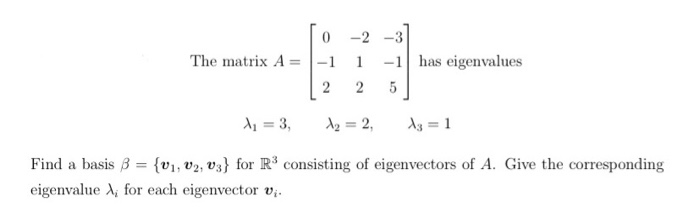0 -2 - The matrix A -11 2 2 -1 has eigenvalues 5 X = 3, A2 = 2, 13 = 1 Find a basis B = {V1, V2, v3} for R3 consisting of eigenvectors of A. Give the corresponding eigenvalue for each eigenvector vi.

• ### please help !!!! 10. 20 points Consider the homogeneous system x' Ax, where 4 0 0 A 1 0 2 02 3 a) Show that v = | 1 | and w = 1-2) are eigenvectors of A. b) Identify the defective eigenvalu...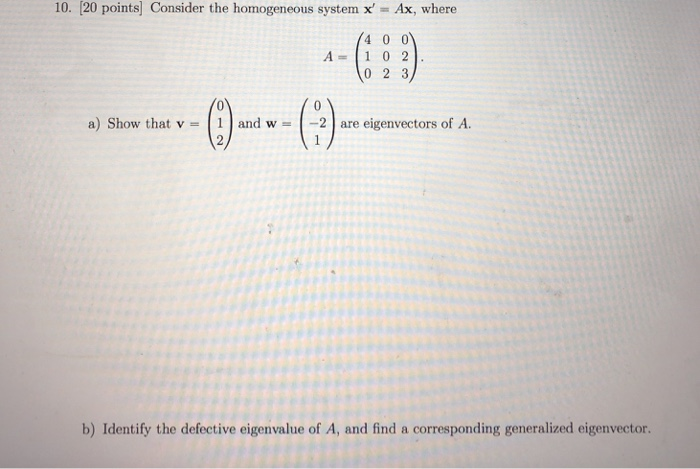please help !!!! 10. 20 points Consider the homogeneous system x' Ax, where 4 0 0 A 1 0 2 02 3 a) Show that v = | 1 | and w = 1-2) are eigenvectors of A. b) Identify the defective eigenvalue of A, and find a corresponding generalized eigenvector Ax c) Write out the general solution of x 10. 20 points Consider the homogeneous system x' Ax, where 4 0 0 A 1 0 2 02 3 a)...

• ### (1 point) Suppose that the matrix A has the following eigenvalues and eigenvectors: 4 = 2...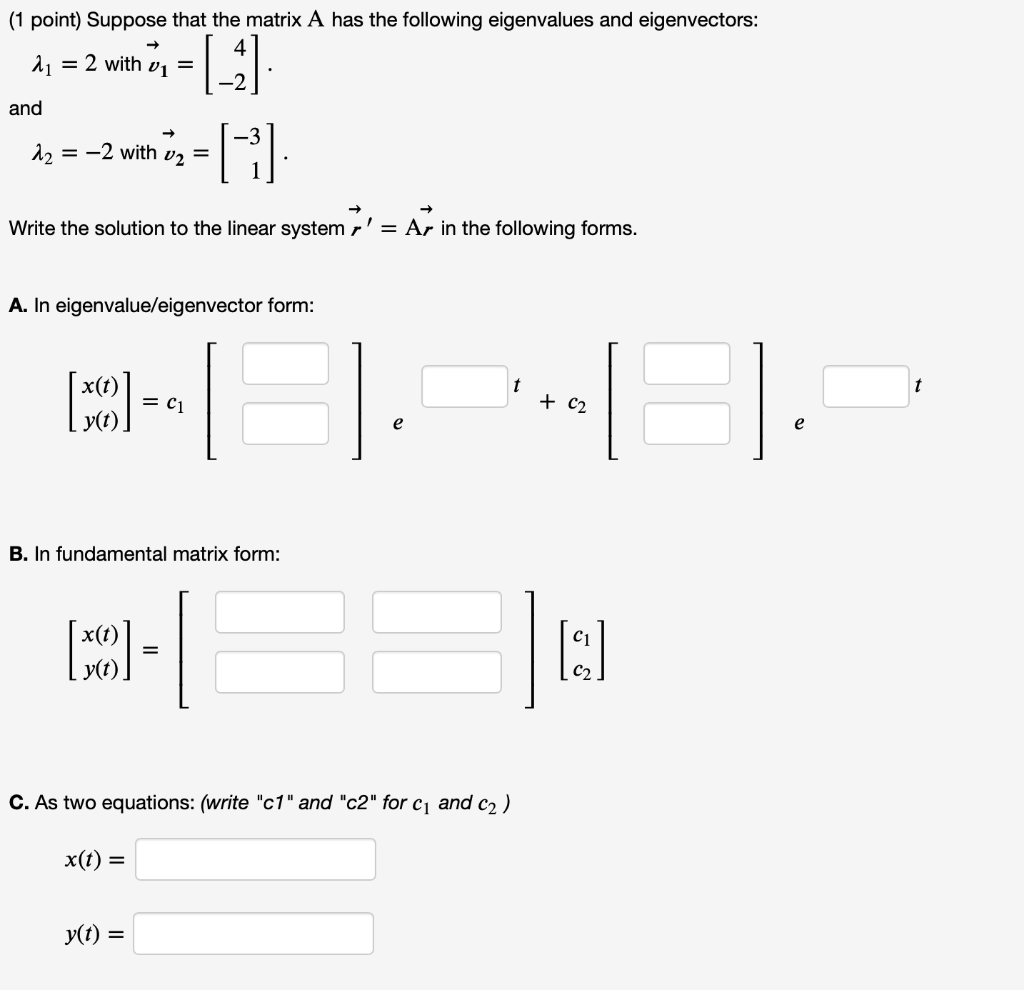(1 point) Suppose that the matrix A has the following eigenvalues and eigenvectors: 4 = 2 with vi = and |_ G 12 = -2 with v2 = Write the solution to the linear system r' = Ar in the following forms. A. In eigenvalue/eigenvector form: x(t) (50) = C1 + C2 e e B. In fundamental matrix form: (MCO) = I: C. As two equations: (write "c1" and "c2" for C1 and c2) x(t) = yt) =

• ### Help with number 1 please! Programming for Math and Science Homework 4 Due by 11:59 p.m. Thursday, May 2, 2019 1. Find the eigenvalues and corresponding eigenvectors for the following matrices sin θ...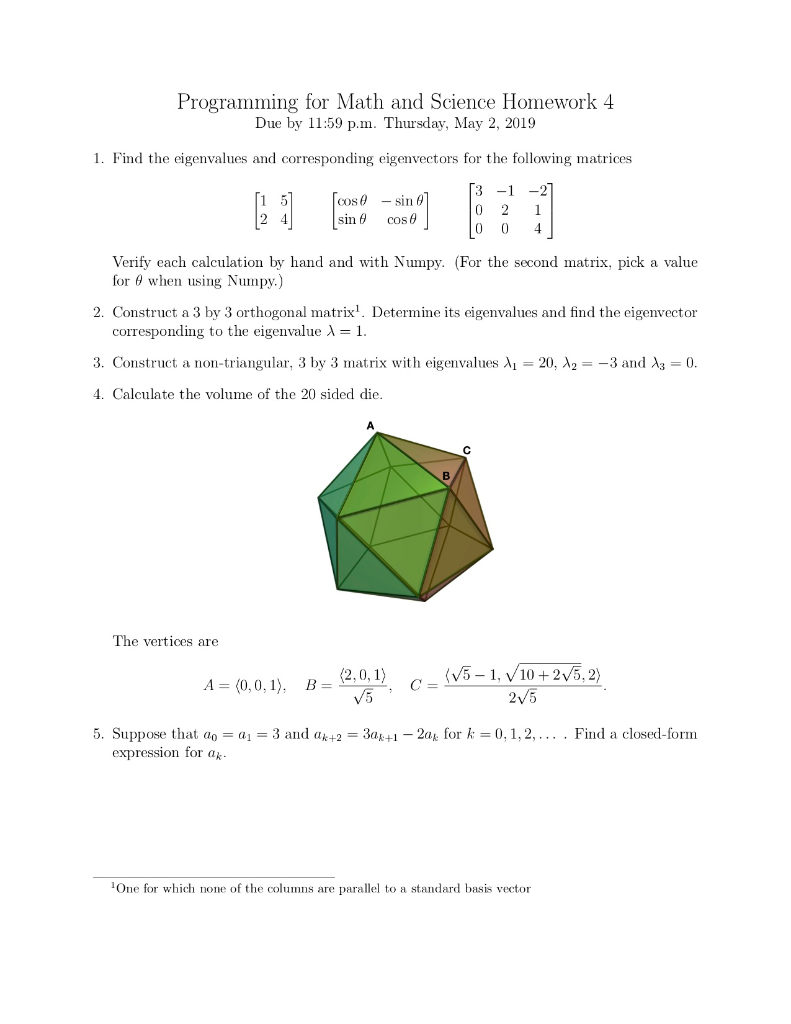Help with number 1 please! Programming for Math and Science Homework 4 Due by 11:59 p.m. Thursday, May 2, 2019 1. Find the eigenvalues and corresponding eigenvectors for the following matrices sin θ cos θ 0 0 4 Verify each calculation by hand and with Numpy. (For the second matrix, pick a value for 0 when using Numpy.) 2. Construct a 3 by 3 orthogonal matrix1. Determine its eigenvalues and find the eigenvector corresponding to the eigenvalue λ-1 3, Construct...

• ### Suppose a dynamical system is modeled with a difference equation x- Ax-1 k0.1.2.3.... where 4 2 8...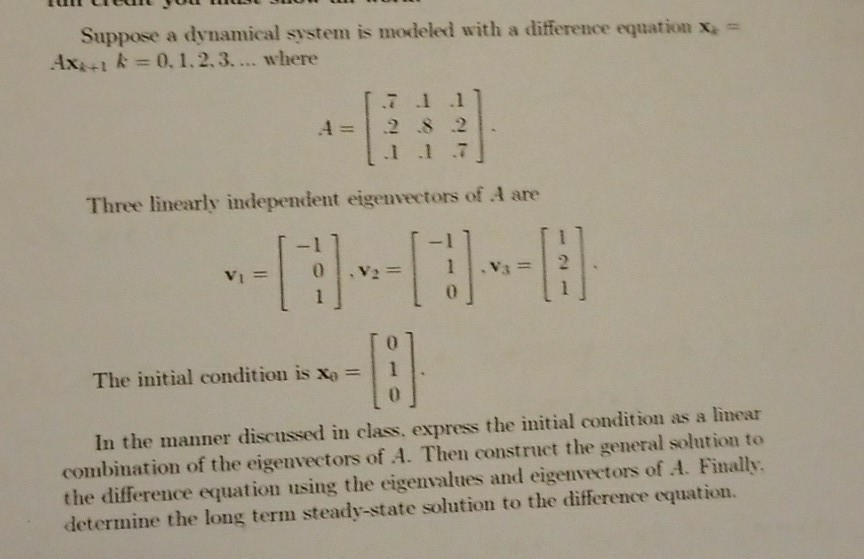Linear Algebra Question Suppose a dynamical system is modeled with a difference equation x- Ax-1 k0.1.2.3.... where 4 2 8 .2 1 1 7 Three linearly independent eigenvectors of A are The initial condition is x: In the manner discussed in class, express the initial condition as a linear combination of the eigenvectors of A. Then construct the general solution to the difference equation using the eigenvalues and eigenvectors of A. Finally determine the long term steady-state solution to the...

• ### Question B3 (10 marks) Solve the following homogeneous system of first order ODE dai di da dt x,(0)=2. Makesures ou usethe initial with the initial conditions (0):0 0)=1, is in the following for...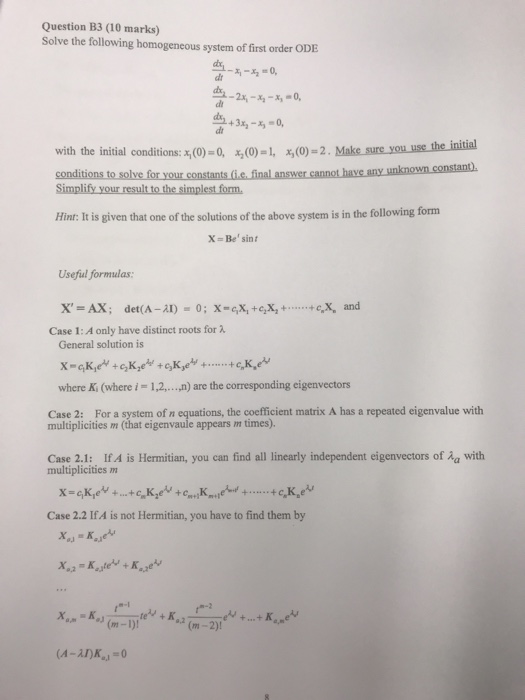Question B3 (10 marks) Solve the following homogeneous system of first order ODE dai di da dt x,(0)=2. Makesures ou usethe initial with the initial conditions (0):0 0)=1, is in the following form Hint: It is given that one of the solutions of the above system Useful formulas Case 1: A only have distinct roots for λ General solution is ,n) are the coresponding eigenvectors where K, (where i = 1,2, Case 2: For a system of n equations, the...

Free Homework App